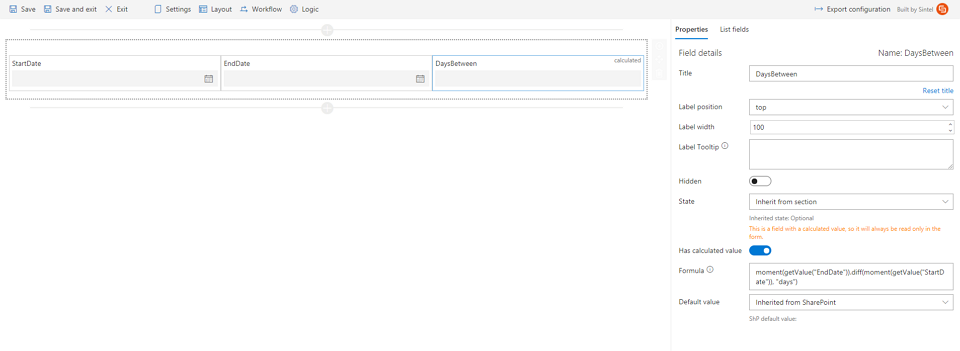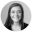Home » Blog » How To: Calculate the number of days between two Date fields on a form# How To: Calculate the number of days between two Date fields on a form#### Michał GołkaSintel Forms allows setting fields values based on formulas which are executed dynamically on a form while changing other field values (refer to calculated fields).
One of the interesting use cases is calculating the number of days between 2 dates.
In our demo list we created three columns:

1. StartDate: type Date and Time
2. EndDate: type Date and Time
3. DaysBetween: type Number

The next step is to open the Sintel Forms Designer and place the new fields on a from.
The last step is to define a calculation formula for the DaysBetween field which requires following steps:

1. Select the field in the Sintel Forms Designer
2. In the “Properties” box enable “Has calculated value” setting
3. In the Formula field insert the following code:
`moment(getValue("EndDate")).diff(moment(getValue("StartDate")), "days")`

Please note that Sintel Forms allows using the momentjs library in formulas.Amy Dermody